Eric Smith

Systems of Linear Equations

Slide Duration:

Section 1: Properties of Real Numbers
Basic Types of Numbers

30m 41s

Intro
0:00
Objectives
0:07
Basic Types of Numbers
0:36
Natural Numbers
1:02
Whole Numbers
1:29
Integers
2:04
Rational Numbers
2:38
Irrational Numbers
5:06
Imaginary Numbers
6:48
Basic Types of Numbers Cont.
8:09
The Big Picture
8:10
Real vs. Imaginary Numbers
8:30
Rational vs. Irrational Numbers
8:48
Basic Types of Numbers Cont.
10:55
Number Line
11:06
Absolute Value
11:44
Inequalities
12:39
Example 1
13:16
Example 2
17:30
Example 3
21:56
Example 4
24:27
Example 5
27:48
Operations on Numbers

19m 26s

Intro
0:00
Objectives
0:06
Operations on Numbers
0:25
0:53
Subtraction
1:33
Multiplication & Division
2:19
Exponents
3:24
Bases
4:04
Square Roots
4:59
Principle Square Roots
5:09
Perfect Squares
6:32
Simplifying and Combining Roots
6:52
Example 1
8:16
Example 2
12:30
Example 3
14:02
Example 4
16:27
Order of Operations

12m 6s

Intro
0:00
Objectives
0:06
The Order of Operations
0:25
Work Inside Parentheses
0:42
Simplify Exponents
0:52
Multiplication & Division from Left to Right
0:57
Addition & Subtraction from Left to Right
1:11
Remember PEMDAS
1:21
The Order of Operations Cont.
2:27
Example
2:43
Example 1
3:55
Example 2
5:36
Example 3
7:35
Example 4
8:56
Properties of Real Numbers

18m 52s

Intro
0:00
Objectives
0:07
The Properties of Real Numbers
0:23
Commutative Property of Addition and Multiplication
0:44
Associative Property of Addition and Multiplication
1:50
Distributive Property of Multiplication Over Addition
3:20
Division Property of Zero
4:46
Division Property of One
5:23
Multiplication Property of Zero
5:56
Multiplication Property of One
6:17
6:29
Why Are These Properties Important?
6:53
Example 1
9:16
Example 2
13:04
Example 3
14:30
Example 4
16:57
Section 2: Linear Equations
The Vocabulary of Linear Equations

12m 22s

Intro
0:00
Objectives
0:09
The Vocabulary of Linear Equations
0:44
Variables
0:52
Terms
1:09
Coefficients
1:40
Like Terms
2:18
Examples of Like Terms
2:37
Expressions
4:01
Equations
4:26
Linear Equations
5:04
Solutions
5:55
Example 1
6:16
Example 2
7:16
Example 3
8:45
Example 4
10:20
Solving Linear Equations in One Variable

28m 52s

Intro
0:00
Objectives
0:08
Solving Linear Equations in One Variable
0:34
Conditional Cases
0:51
Identity Cases
1:09
1:30
Solving Linear Equations in One Variable Cont.
2:00
2:10
Multiplication Property of Equality
2:43
Steps to Solve Linear Equations
3:14
Example 1
4:22
Example 2
8:21
Example 3
12:32
Example 4
14:19
Example 5
17:25
Example 6
22:17
Solving Formulas

12m 2s

Intro
0:00
Objectives
0:06
Solving Formulas
0:18
Formulas
0:26
Use the Same Properties as Solving Linear Equations
1:36
1:55
Multiplication Property of Equality
1:58
Steps to Solve Formulas
2:43
Example 1
3:56
Example 2
6:09
Example 3
8:39
Applications of Linear Equations

28m 41s

Intro
0:00
Objectives
0:10
Applications of Linear Equations
0:43
The Six-Step Method to Solving Word Problems
0:55
Common Terms
3:12
Example 1
5:03
Example 2
9:40
Example 3
13:48
Example 4
17:58
Example 5
23:28
Applications of Linear Equations, Motion & Mixtures

24m 26s

Intro
0:00
Objectives
0:21
Motion and Mixtures
0:46
Motion Problems: Distance, Rate, and Time
1:06
Mixture Problems: Amount, Percent, and Total
1:27
The Table Method
1:58
The Beaker Method
3:38
Example 1
5:05
Example 2
9:44
Example 3
14:20
Example 4
19:13
Section 3: Graphing
Rectangular Coordinate System

22m 55s

Intro
0:00
Objectives
0:11
The Rectangular Coordinate System
0:39
The Cartesian Coordinate System
0:40
X-Axis
0:54
Y-Axis
1:04
Origin
1:11
1:26
Ordered Pairs
2:10
Example 1
2:55
The Rectangular Coordinate System Cont.
6:09
X-Intercept
6:45
Y-Intercept
6:55
Relation of X-Values and Y-Values
7:30
Example 2
11:03
Example 3
12:13
Example 4
14:10
Example 5
18:38
Slope & Graphing

27m 58s

Intro
0:00
Objectives
0:11
Slope and Graphing
0:48
Standard Form
1:14
Example 1
2:24
Slope and Graphing Cont.
4:58
Slope, m
5:07
Slope is Rise over Run
6:11
Don't Mix Up the Coordinates
8:20
Example 2
9:39
Slope and Graphing Cont.
14:26
Slope-Intercept Form
14:34
Example 3
16:55
Example 4
18:00
Slope and Graphing Cont.
19:00
Rewriting an Equation in Slope-Intercept Form
19:39
Rewriting an Equation in Standard Form
20:09
Slopes of Vertical & Horizontal Lines
20:56
Example 5
22:49
Example 6
24:09
Example 7
25:59
Example 8
26:57
Linear Equations in Two Variables

20m 36s

Intro
0:00
Objectives
0:13
Linear Equations in Two Variables
0:36
Point-Slope Form
1:07
Substitute in the Point and the Slope
2:21
Parallel Lines: Two Lines with the Same Slope
4:05
Perpendicular Lines: Slopes are Negative Reciprocals of Each Other
4:39
Perpendicular Lines: Product of Slopes is -1
5:24
Example 1
6:02
Example 2
7:50
Example 3
10:49
Example 4
13:26
Example 5
15:30
Example 6
17:43
Section 4: Functions
Introduction to Functions

21m 24s

Intro
0:00
Objectives
0:07
Introduction to Functions
0:58
Relations
1:03
Functions
1:37
Independent Variables
2:00
Dependent Variables
2:11
Function Notation
2:21
Function
3:43
Input and Output
3:53
Introduction to Functions Cont.
4:45
Domain
4:46
Range
4:55
Functions Represented by a Diagram
6:41
Natural Domain
9:11
Evaluating Functions
12:02
Example 1
13:13
Example 2
15:03
Example 3
16:18
Example 4
19:54
Graphing Functions

16m 12s

Intro
0:00
Objectives
0:09
Graphing Functions
0:54
Using Slope-Intercept Form
1:56
Vertical Line Test
2:58
Determining the Domain
4:20
Determining the Range
5:43
Example 1
6:06
Example 2
7:18
Example 3
8:31
Example 4
11:04
Section 5: Systems of Linear Equations
Systems of Linear Equations

25m 54s

Intro
0:00
Objectives
0:13
Systems of Linear Equations
0:46
System of Equations
0:51
System of Linear Equations
1:15
Solutions
1:35
Points as Solutions
1:53
Finding Solutions Graphically
5:13
Example 1
6:37
Example 2
12:07
Systems of Linear Equations Cont.
17:01
One Solution, No Solution, or Infinite Solutions
17:10
Example 3
18:31
Example 4
22:37
Solving a System Using Substitution

20m 1s

Intro
0:00
Objectives
0:09
Solving a System Using Substitution
0:32
Substitution Method
1:24
Substitution Example
2:35
One Solution, No Solution, or Infinite Solutions
7:50
Example 1
9:45
Example 2
12:48
Example 3
15:01
Example 4
17:30
Solving a System Using Elimination

19m 40s

Intro
0:00
Objectives
0:09
Solving a System Using Elimination
0:27
Elimination Method
0:42
Elimination Example
2:01
One Solution, No Solution, or Infinite Solutions
7:05
Example 1
8:53
Example 2
11:46
Example 3
15:37
Example 4
17:45
Applications of Systems of Equations

24m 34s

Intro
0:00
Objectives
0:12
Applications of Systems of Equations
0:30
Word Problems
1:31
Example 1
2:17
Example 2
7:55
Example 3
13:07
Example 4
17:15
Section 6: Inequalities
Solving Linear Inequalities in One Variable

17m 13s

Intro
0:00
Objectives
0:08
Solving Linear Inequalities in One Variable
0:37
Inequality Expressions
0:46
Linear Inequality Solution Notations
3:40
Inequalities
3:51
Interval Notation
4:04
Number Lines
4:43
Set Builder Notation
5:24
Use Same Techniques as Solving Equations
6:59
'Flip' the Sign when Multiplying or Dividing by a Negative Number
7:12
'Flip' Example
7:50
Example 1
8:54
Example 2
11:40
Example 3
14:01
Compound Inequalities

16m 13s

Intro
0:00
Objectives
0:07
Compound Inequalities
0:37
'And' vs. 'Or'
0:44
'And'
3:24
'Or'
3:35
'And' Symbol, or Intersection
3:51
'Or' Symbol, or Union
4:13
Inequalities
4:41
Example 1
6:22
Example 2
9:30
Example 3
11:27
Example 4
13:49
Solving Equations with Absolute Values

14m 12s

Intro
0:00
Objectives
0:08
Solve Equations with Absolute Values
0:18
Solve Equations with Absolute Values Cont.
1:11
Steps to Solving Equations with Absolute Values
2:21
Example 1
3:23
Example 2
6:34
Example 3
10:12
Inequalities with Absolute Values

17m 7s

Intro
0:00
Objectives
0:07
Inequalities with Absolute Values
0:23
Recall…
2:08
Example 1
3:39
Example 2
6:06
Example 3
8:14
Example 4
10:29
Example 5
13:29
Graphing Inequalities in Two Variables

15m 33s

Intro
0:00
Objectives
0:07
Graphing Inequalities in Two Variables
0:32
Split Graph into Two Regions
1:53
Graphing Inequalities
5:44
Test Points
6:20
Example 1
7:11
Example 2
10:17
Example 3
13:06
Systems of Inequalities

21m 13s

Intro
0:00
Objectives
0:08
Systems of Inequalities
0:24
Test Points
1:10
Steps to Solve Systems of Inequalities
1:25
Example 1
2:23
Example 2
7:28
Example 3
12:51
Section 7: Polynomials
Integer Exponents

44m 51s

Intro
0:00
Objectives
0:09
Integer Exponents
0:42
Exponents 'Package' Multiplication
1:25
Example 1
2:00
Example 2
3:13
Integer Exponents Cont.
4:50
Product Rule for Exponents
4:51
Example 3
7:16
Example 4
10:15
Integer Exponents Cont.
13:13
Power Rule for Exponents
13:14
Power Rule with Multiplication and Division
15:33
Example 5
16:18
Integer Exponents Cont.
20:04
Example 6
20:41
Integer Exponents Cont.
25:52
Zero Exponent Rule
25:53
Quotient Rule
28:24
Negative Exponents
30:14
Negative Exponent Rule
32:27
Example 7
34:05
Example 8
36:15
Example 9
39:33
Example 10
43:16

18m 33s

Intro
0:00
Objectives
0:07
0:25
Terms
0:33
Coefficients
0:51
1:13
Like Terms
1:29
Polynomials
2:21
Monomials, Binomials, Trinomials, and Polynomials
5:41
Degrees
7:00
Evaluating Polynomials
8:12
9:25
Example 1
11:48
Example 2
13:00
Example 3
14:41
Example 4
16:15
Multiplying Polynomials

25m 7s

Intro
0:00
Objectives
0:06
Multiplying Polynomials
0:41
Distributive Property
1:00
Example 1
2:49
Multiplying Polynomials Cont.
8:22
Organize Terms with a Table
8:23
Example 2
13:40
Multiplying Polynomials Cont.
16:33
Multiplying Binomials with FOIL
16:48
Example 3
18:49
Example 4
20:04
Example 5
21:42
Dividing Polynomials

44m 56s

Intro
0:00
Objectives
0:07
Dividing Polynomials
0:29
Dividing Polynomials by Monomials
2:10
Dividing Polynomials by Polynomials
2:59
Dividing Numbers
4:09
Dividing Polynomials Example
8:39
Example 1
12:35
Example 2
14:40
Example 3
16:45
Example 4
21:13
Example 5
24:33
Example 6
29:02
Dividing Polynomials with Synthetic Division Method
33:36
Example 7
38:43
Example 8
42:24
Section 8: Factoring Polynomials
Greatest Common Factor & Factor by Grouping

28m 27s

Intro
0:00
Objectives
0:09
Greatest Common Factor
0:31
Factoring
0:40
Greatest Common Factor (GCF)
1:48
GCF for Polynomials
3:28
Factoring Polynomials
6:45
Prime
8:21
Example 1
9:14
Factor by Grouping
14:30
Steps to Factor by Grouping
17:03
Example 2
17:43
Example 3
19:20
Example 4
20:41
Example 5
22:29
Example 6
26:11
Factoring Trinomials

21m 44s

Intro
0:00
Objectives
0:06
Factoring Trinomials
0:25
Recall FOIL
0:26
Factor a Trinomial by Reversing FOIL
1:52
Tips when Using Reverse FOIL
5:31
Example 1
7:04
Example 2
9:09
Example 3
11:15
Example 4
13:41
Factoring Trinomials Cont.
15:50
Example 5
18:42
Factoring Trinomials Using the AC Method

30m 9s

Intro
0:00
Objectives
0:08
Factoring Trinomials Using the AC Method
0:27
Factoring when Leading Term has Coefficient Other Than 1
1:07
Reversing FOIL
1:18
Example 1
1:46
Example 2
4:28
Factoring Trinomials Using the AC Method Cont.
7:45
The AC Method
8:03
Steps to Using the AC Method
8:19
Tips on Using the AC Method
9:29
Example 3
10:45
Example 4
16:50
Example 5
21:08
Example 6
24:58
Special Factoring Techniques

30m 14s

Intro
0:00
Objectives
0:07
Special Factoring Techniques
0:26
Difference of Squares
1:46
Perfect Square Trinomials
2:38
No Sum of Squares
3:32
Special Factoring Techniques Cont.
4:03
Difference of Squares Example
4:04
Perfect Square Trinomials Example
5:29
Example 1
7:31
Example 2
9:59
Example 3
11:47
Example 4
15:09
Special Factoring Techniques Cont.
19:07
Sum of Cubes and Difference of Cubes
19:08
Example 5
23:13
Example 6
26:12

23m 38s

Intro
0:00
Objectives
0:08
0:19
0:20
Zero Factor Property
1:39
Zero Factor Property Example
2:34
Example 1
4:00
Solving Quadratic Equations by Factoring Cont.
5:54
Example 2
7:28
Example 3
11:09
Example 4
14:22
Solving Quadratic Equations by Factoring Cont.
18:17
Higher Degree Polynomial Equations
18:18
Example 5
20:22

29m 27s

Intro
0:00
Objectives
0:12
0:29
Linear Factors
0:38
1:22
Principle of Square Roots
3:36
Completing the Square
4:50
Steps for Using Completing the Square
5:15
Completing the Square Works on All Quadratic Equations
6:41
7:28
Discriminants
8:25
10:11
Example 1
11:54
Example 2
13:03
Example 3
16:30
Example 4
21:29
Example 5
25:07

16m 47s

Intro
0:00
Objectives
0:08
0:24
Using a Substitution
0:53
U-Substitution
1:26
Example 1
2:07
Example 2
5:36
Example 3
8:31
Example 4
11:14

29m 4s

Intro
0:00
Objectives
0:09
0:35
Squared Variable
0:40
Principle of Square Roots
0:51
Example 1
1:09
Example 2
2:04
3:34
Example 3
4:42
Example 4
13:33
Example 5
20:50

26m 53s

Intro
0:00
Objectives
0:06
0:39
Axis of Symmetry
1:46
Vertex
2:12
Transformations
2:57
3:23
Example 1
5:06
Example 2
6:02
Example 3
9:07
11:26
Completing the Square
12:02
Vertex Shortcut
12:16
Example 4
13:49
Example 5
17:25
Example 6
20:07
Example 7
23:43
Polynomial Inequalities

21m 42s

Intro
0:00
Objectives
0:07
Polynomial Inequalities
0:30
Solving Polynomial Inequalities
1:20
Example 1
2:45
Polynomial Inequalities Cont.
5:12
Larger Polynomials
5:13
Positive or Negative Intervals
7:16
Example 2
9:01
Example 3
13:53
Section 10: Rational Equations
Multiply & Divide Rational Expressions

26m 41s

Intro
0:00
Objectives
0:09
Multiply and Divide Rational Expressions
0:44
Rational Numbers
0:55
Dividing by Zero
1:45
Canceling Extra Factors
2:43
Negative Signs in Fractions
4:52
Multiplying Fractions
6:26
Dividing Fractions
7:17
Example 1
8:04
Example 2
14:01
Example 3
16:23
Example 4
18:56
Example 5
22:43

20m 24s

Intro
0:00
Objectives
0:07
0:41
Common Denominators
0:52
Common Denominator Examples
1:14
Steps to Adding and Subtracting Rational Expressions
2:39
Example 1
3:34
Example 2
5:27
Adding and Subtracting Rational Expressions Cont.
6:57
Least Common Denominators
6:58
Transitioning from Fractions to Rational Expressions
9:08
Identifying Least Common Denominators for Rational Expressions
9:56
10:41
Example 3
11:19
Example 4
12:36
Example 5
15:08
Example 6
16:46
Complex Fractions

18m 23s

Intro
0:00
Objectives
0:09
Complex Fractions
0:37
Dividing to Simplify Complex Fractions
1:10
Example 1
2:03
Example 2
3:58
Complex Fractions Cont.
9:15
Using the Least Common Denominator to Simplify Complex Fractions
9:16
10:07
Example 3
10:42
Example 4
14:28
Solving Rational Equations

16m 24s

Intro
0:00
Objectives
0:07
Solving Rational Equations
0:23
Isolate the Specified Variable
1:23
Example 1
1:58
Example 2
5:00
Example 3
8:23
Example 4
13:25
Rational Inequalities

18m 54s

Intro
0:00
Objectives
0:06
Rational Inequalities
0:18
Testing Intervals for Rational Inequalities
0:38
Steps to Solving Rational Inequalities
1:05
Tips to Solving Rational Inequalities
2:27
Example 1
3:33
Example 2
12:21
Applications of Rational Expressions

20m 20s

Intro
0:00
Objectives
0:07
Applications of Rational Expressions
0:27
Work Problems
1:05
Example 1
2:58
Example 2
6:45
Example 3
13:17
Example 4
16:37
Variation & Proportion

27m 4s

Intro
0:00
Objectives
0:10
Variation and Proportion
0:34
Variation
0:35
Inverse Variation
1:01
Direct Variation
1:10
Setting Up Proportions
1:31
Example 1
2:27
Example 2
5:36
Variation and Proportion Cont.
8:29
Inverse Variation
8:30
Example 3
9:20
Variation and Proportion Cont.
12:41
Constant of Proportionality
12:42
Example 4
13:59
Variation and Proportion Cont.
16:17
Varies Directly as the nth Power
16:30
Varies Inversely as the nth Power
16:53
Varies Jointly
17:09
Combining Variation Models
17:36
Example 5
19:09
Example 6
22:10
Rational Exponents

14m 32s

Intro
0:00
Objectives
0:07
Rational Exponents
0:32
Power on Top, Root on Bottom
1:05
Example 1
1:37
Rational Exponents Cont.
4:04
Using Rules from Exponents for Radicals as Exponents
4:05
Combining Terms Under a Single Root
4:50
Example 2
5:21
Example 3
7:39
Example 4
11:23
Example 5
13:14
Simplify Rational Exponents

15m 12s

Intro
0:00
Objectives
0:07
Simplify Rational Exponents
0:25
0:26
Product Rule to Simplify Square Roots
1:11
1:42
Applications of Product and Quotient Rules
2:17
Higher Roots
2:48
Example 1
3:39
Example 2
6:35
Example 3
8:41
Example 4
11:09

17m 22s

Intro
0:00
Objectives
0:07
0:33
Like Terms
1:29
Bases and Exponents May be Different
2:02
Bases and Powers Must be Same when Adding and Subtracting
2:42
3:55
Example 1
4:47
Example 2
6:00
7:10
Simplify the Bases to Look the Same
7:25
Example 3
8:23
Example 4
11:45
Example 5
15:10

19m 24s

Intro
0:00
Objectives
0:08
0:25
0:26
1:11
Don’t Distribute Powers
2:54
4:25
Rationalizing Denominators
6:40
Example 1
7:22
Example 2
8:32
9:23
Rationalizing Denominators with Higher Roots
9:25
Example 3
10:51
Example 4
11:53
13:13
Rationalizing Denominators with Conjugates
13:14
Example 5
15:52
Example 6
17:25

15m 5s

Intro
0:00
Objectives
0:07
0:17
0:18
Isolate the Roots and Raise to Power
0:34
Example 1
1:13
Example 2
3:09
7:04
7:05
Example 3
7:54
Example 4
13:07
Complex Numbers

29m 16s

Intro
0:00
Objectives
0:06
Complex Numbers
1:05
Imaginary Numbers
1:08
Complex Numbers
2:27
Real Parts
2:48
Imaginary Parts
2:51
Commutative, Associative, and Distributive Properties
3:35
4:04
Multiplying Complex Numbers
6:16
Dividing Complex Numbers
8:59
Complex Conjugate
9:07
Simplifying Powers of i
14:34
Shortcut for Simplifying Powers of i
18:33
Example 1
21:14
Example 2
22:15
Example 3
23:38
Example 4
26:33
Bookmark & Share Embed

## Copy & Paste this embed code into your website’s HTML

Please ensure that your website editor is in text mode when you paste the code.
(In Wordpress, the mode button is on the top right corner.)
×
• - Allow users to view the embedded video in full-size.
Since this lesson is not free, only the preview will appear on your website.

• ## Related Books0 answersPost by Rose A on February 19, 2018Some of the practice questions are wrong...It's not you that does the pracice questions from what I understood?... who can I contact for issues with the practice questions!?1 answerLast reply by: Professor Eric SmithThu Dec 31, 2015 5:32 PMPost by Bongani Makhathini on December 30, 2015I believe that example 3 does have a solution, its just that the lines will across outside the graphing paper displayed on the screen as the lines progress. I came to this conclusion because the points 4, 2 and 6 are even numbers. However, 3 is an odd number, tilting the line just slightly anti-clockwise. :-)0 answersPost by Farhat Muruwat on March 24, 2014Step 3. 2y âˆ’ x = 3 â†’ ( 0,[3/2] ),( âˆ’ [3/2],0 )How did you get 2y - x = 3?0 answersPost by Farhat Muruwat on March 24, 2014Q. Solving the system by graphingy âˆ’ x = 42y âˆ’ x = 3Step 1. Find two points for each equation by setting x = 0 then y = 0Step 2. y âˆ’ x = 4 â†’ ( 0,4 ),( âˆ’ 4,0 )How did you get y - x = 4?

### Systems of Linear Equations

• A system of equations is made from a pair or more equations put together. If all the equations are lines, it is said to be a linear system.
• A solution to a system must make all equations true.
• To solve a system graphically, you plot out each equation and identify where they cross. For this method to work you must draw the graphs accurately.
• A system in two variables could have one solution, no solution, or infinite solutions. You can recognize these by the lines either crossing at one point, being parallel, or being on top of one another.

### Systems of Linear Equations

Solving the system by graphing
x + 2y = 3
5x + y = 5
• Find two points for each equation by setting x = 0 then y = 0
• x + 2y = 3 → ( 0,[1/2] ),( 3,0 )
• 5x + y = 5 → ( 5.0, ),( 5,0 )
Graph to find intersection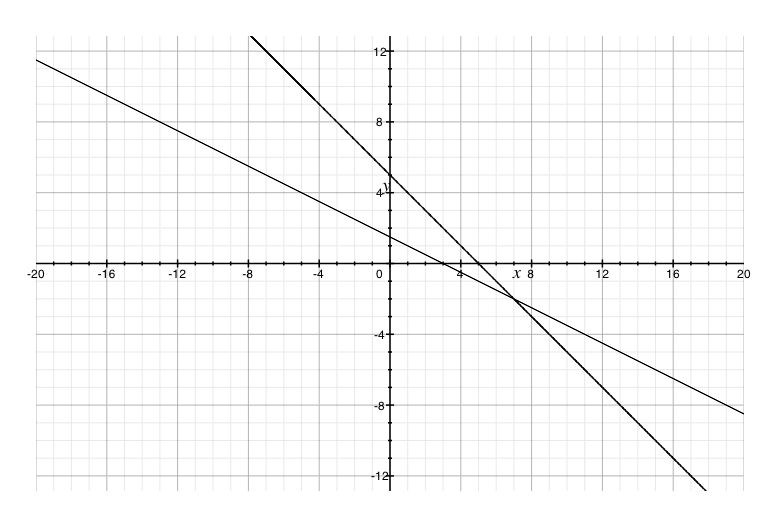(7, - 2)
Solving the system by graphing
y - x = 4
2y - x = 3
• Find two points for each equation by setting x = 0 then y = 0
• y − x = 4 → ( 0,4 ),( − 4,0 )
• 2y − x = 3 → ( 0,[3/2] ),( − [3/2],0 )
Graph to find intersection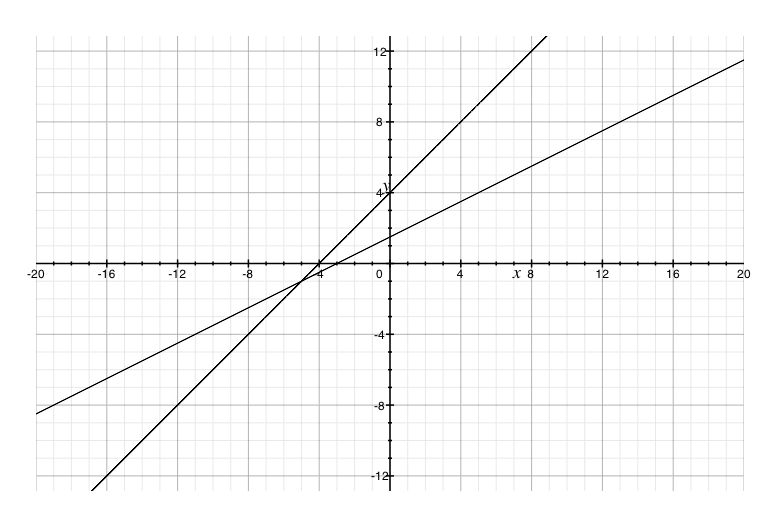( - 5, - 1)
Solving the system by graphing
- x + 4y = 20
y - 3x = 16
• Find two points for each equation by setting x = 0 then y = 0
• x + 4y = 20 → ( 0,5 ),( − 20,0 )
• y − 3x = 16 → ( 0, − [16/3] ),( 16,0 )
Graph to find intersection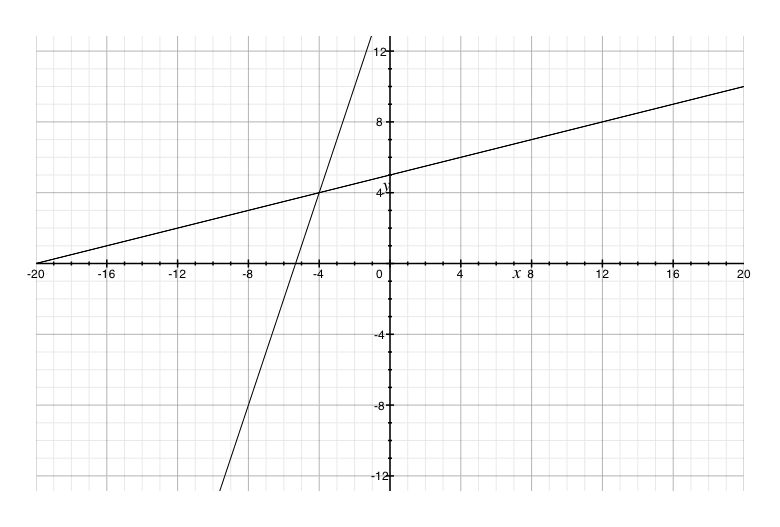( - 4,4)
Solving the system by graphing
- x + 6y = 12
y + x = 9
• Find two points for each equation by setting x = 0 then y = 0
• − x + 6y = 12 → ( 0, − 12 ),( 2,0 )
• y + x = 9 → ( 9,0 ),( 0,9 )
Graph to find intersection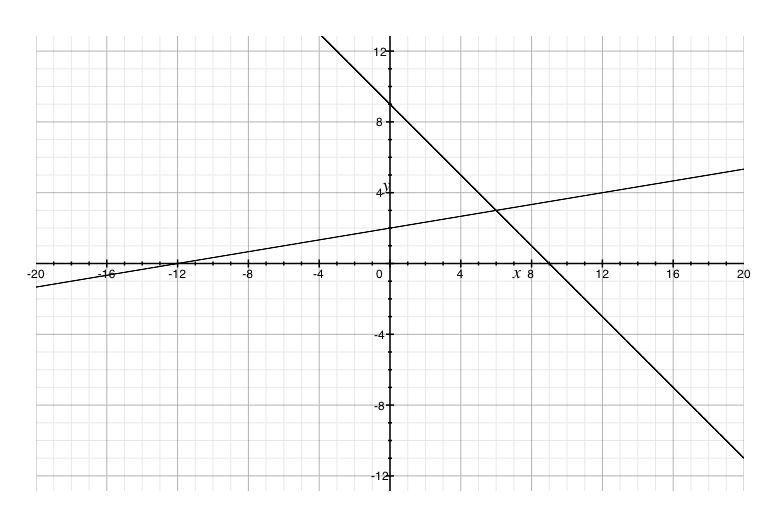(6,3)
Solving the system by graphing
x + 2y = 16
x + y = 8
• Find two points for each equation by setting x = 0 then y = 0
• x + 2y = 16 → ( 0,8 ),( 16,0 )
• x − y = 8 → ( 8,0 ),( 0,8 )
Graph to find intersection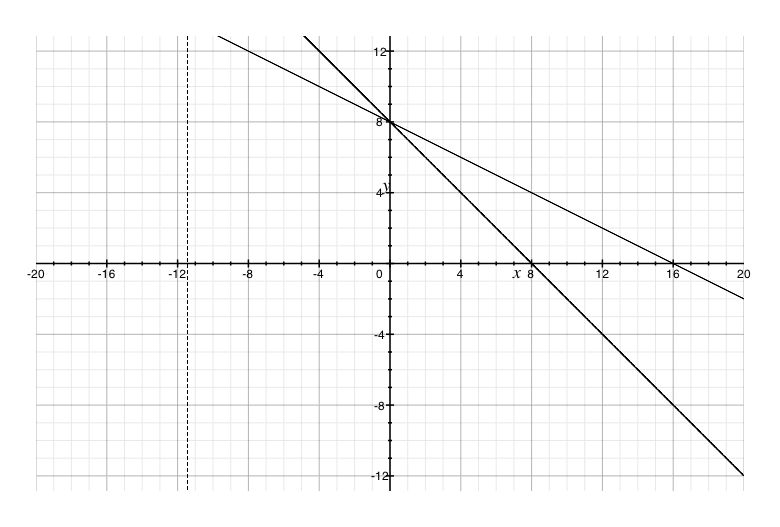(0,8)
Solving the system by graphing
x + 2y = - 12
3y - x = 6
• Find two points for each equation by setting x = 0 then y = 0
• x + 2y = − 12 → ( − 12,0 ),( 0, − 6 )
• 3y − x = 6 → ( − 6,0 ),( 0,2 )
Graph to find intersection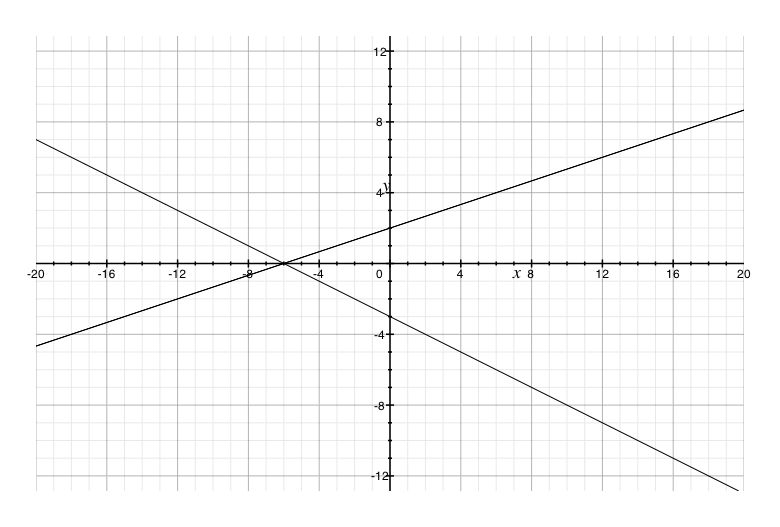( - 6,0)
Solving the system by graphing
x + y = 5
y = - 3
• Find two points for each equation by setting x = 0 then y = 0
• x + y = 5 → ( 5,0 ),( 0,5 )
• y = − 3 → ( 0, − 3 ),( 0, − 3 )
Graph to find intersection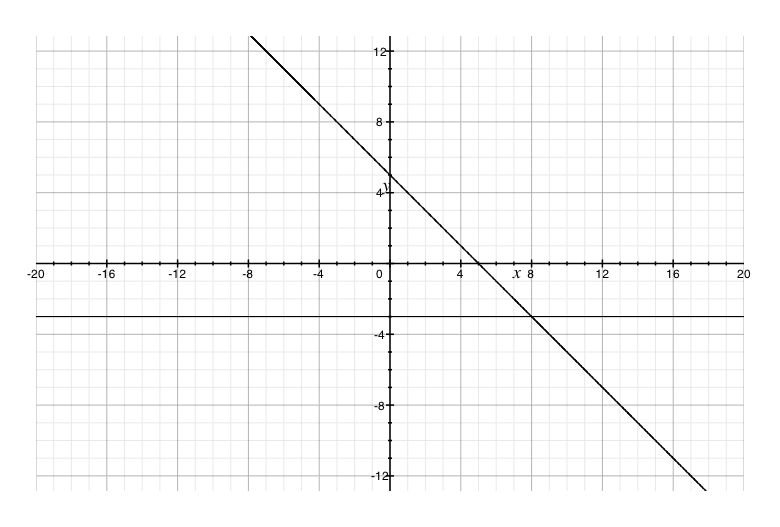(8, - 3)
Solving the system by graphing
y - 3x = 2
- 9x + 3y = 6
• Find two points for each equation by setting x = 0 then y = 0
• x + y = 5 → ( − [3/2],0 ),( 0,2 )
• y = − 3 → ( − [3/2],0 ),( 0,2 )
Graph to find intersection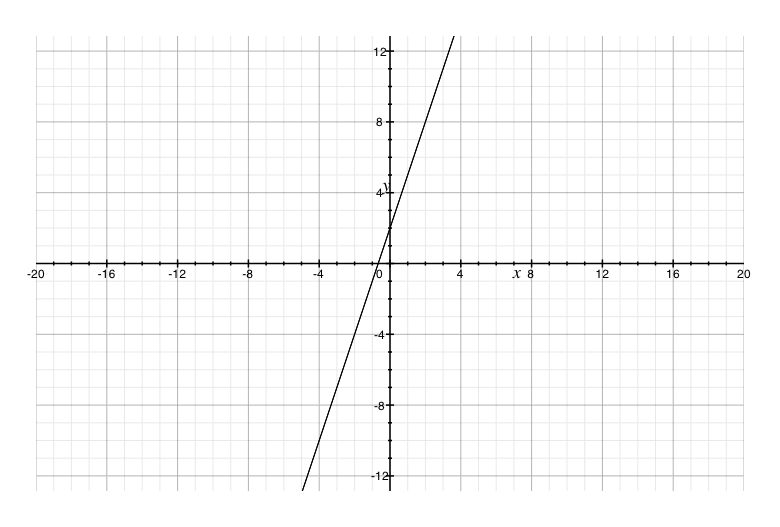Infinite solutions
Solving the system by graphing
2y - x = - 8
y - x = - 3
• Find two points for each equation by setting x = 0 then y = 0
• 2y − x = − 8 → ( 0, − 4 ),( 8,0 )
• − y + x = 3 → ( 3,0 ),( 0, − 3 )
Graph to find intersection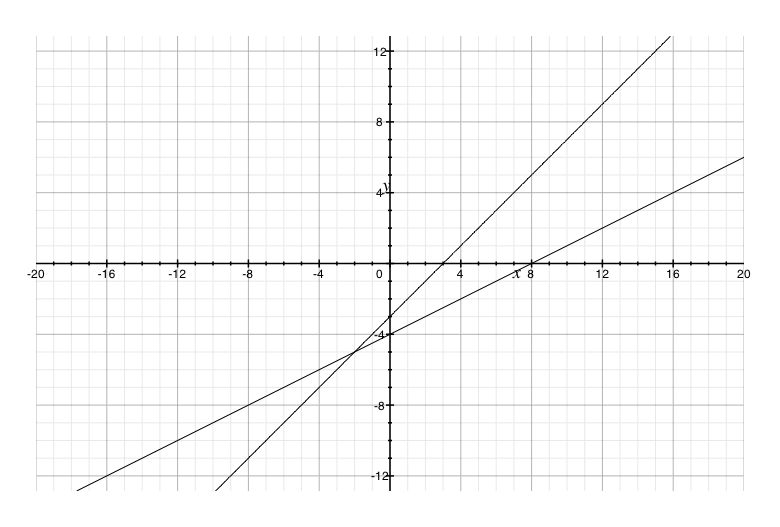( - 2, - 5)
Solving the system by graphing
y + 2x = 5
x + [y/2] = 2
• Find two points for each equation by setting x = 0 then y = 0
• y + 2x = 5 → ( 0,5 ),( [5/2],0 )
• x + [y/2] = 2 → ( 2,0 ),( 0,4 )
Graph to find intersection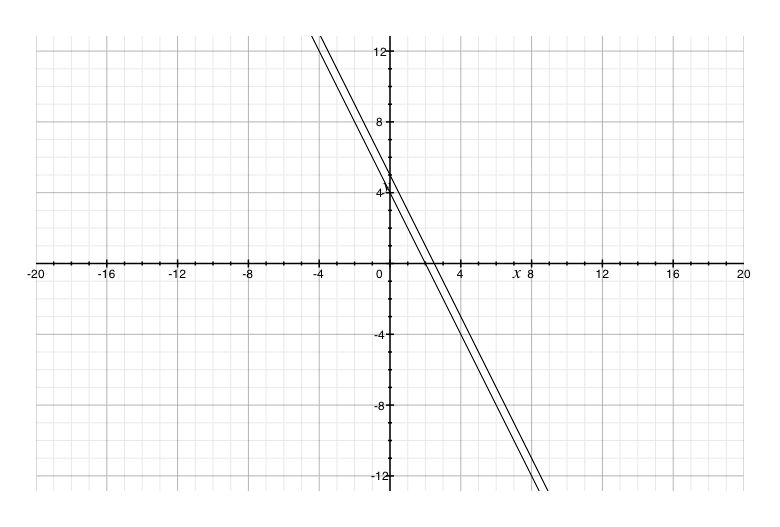No solutions

*These practice questions are only helpful when you work on them offline on a piece of paper and then use the solution steps function to check your answer.

### Systems of Linear Equations

Lecture Slides are screen-captured images of important points in the lecture. Students can download and print out these lecture slide images to do practice problems as well as take notes while watching the lecture.

• Intro 0:00
• Objectives 0:13
• Systems of Linear Equations 0:46
• System of Equations
• System of Linear Equations
• Solutions
• Points as Solutions
• Finding Solutions Graphically
• Example 1 6:37
• Example 2 12:07
• Systems of Linear Equations Cont. 17:01
• One Solution, No Solution, or Infinite Solutions
• Example 3 18:31
• Example 4 22:37

### Transcription: Systems of Linear Equations

Welcome back to www.educator.com.0000

In this lesson we are going to take a look at systems of linear equations, pairs of linear equations0002

and get into their solutions and how we can graph them.0008

More specific things that we want to know is, what exactly is a system of equation and how can we find their solutions?0015

One of the greatest ways that we can do to find their solutions is just looking at their graphs.0023

Some special things that we want to know in terms of their types of solutions is how do we know when there is going to be no solution0030

and how we will know when they will actually be an infinite amount of solutions.0036

Watch out for those two as we get into the nuts and bolts of all this.0041

What is a system of equations?0049

A system is a pair or more of an equation coupled together.0052

Sometimes you will see a { } put onto equations just to show that they are all being combined and coupled together.0059

Here I have a pair, but I could have 3, 4, 5 and many more than just that.0070

Now if all of the equations that are being coupled together are lines, then we will call these linear equations.0076

You will notice in the examples that I have up here, both of these are lines in standard form.0083

I have a system of linear equations.0089

In order to be a solution of a system, it must satisfy all of the equations in that system all simultaneously, all at once.0094

If it only satisfies the first equation then that is not good enough to be a solution of the system, it must satisfy all of them.0101

Let us quickly look at how we can determine if a point is a part of the solution or not.0116

We are going to do this by substituting it into an actual system.0123

My system over here is 2x + 3y = 17 and x + 4y = 21.0126

Is the point 7, 1 a solution or not?0133

Let us go ahead and write down our system.0138

Let us first put it into equation 1, we have (2 × 7) + (3 × 1) is it equal to 17, I do not know.0144

Let us find out.0157

2 × 7 would be 14 + 3 and sure enough I get 17.0158

I know it satisfies the first equation just fine.0168

Let us take it and substitute it into this second equation.0174

I have 7x + (4 × y value × 1).0182

4 × 1 = 4, 7 + 4, that one does not work out because 11 is not equal to 21.0193

Even though it only satisfies one of the equations, I can say that it is not a solution.0206

It only satisfies one equation.0223

Let us try this with the point 1, 5 and let us see if that is a solution of the system.0234

We will put in 2 or 3 if we want to know if this is equal to 17.0241

I will put in 1 for x and we will put in 5 for y and let us simplify it.0248

2 × 1 is 2 ,3 × 5 is 15 and 2 + 15 =17 and this one checks out.0258

Now let us substitute it into the second equation.0273

I will take 1 and put in for x, we will take 5 and put it in for y.0278

I have 1 + 20 this that equal 21? It does 21 equals 21.0287

I can say it is a solution and it satisfies both of the equations.0296

There are few ways that you can go about solving a system of equations and we will see them in later lessons0316

or the first ways that you can sense what the solution should be is to the graph the equations that are present.0321

If these are linear equations that you are dealing with then you can use the techniques of graphing your lines to make this a much easier process.0328

Let us work on finding our solutions graphically.0335

To do this, all you have to do is graph the equations and then find out where those equations cross, when they do that is going to be our solution.0339

There is one downside to this so be very careful.0349

In order for this process to work out, you must make accurate graphs.0353

If your lines are a little wavy or you do not make them very accurate then you might think you know where it cross, but actually identify a different point.0358

Be careful and make accurate graphs.0369

Since finding solutions using a graph is as nice and visual, we definitely will start with it0381

but we will use some of those later techniques for solving because the accuracy does tend to be an issue with the graphs.0388

Let us get into the graphing and finding a solution that way, I will try.0400

Here I have a system of linear equations and it looks like both of them are almost written in standard form.0405

The second one is not quite as in that form because it has a negative sign out front.0412

I think we will be able to graph it just fine.0417

I'm going to graph it by using its intercepts.0419

We will make a little chart for our first equation and I'm going to identify where it crosses the y axis and the x axis by plugging in an appropriate value of 0.0424

If I use 0 in my equation for the first one, I just need to solve and figure out what y is.0436

Putting in a 0, we will limit that term and solving the rest out, divide it by 3 and I will get that y =2.0445

I know that is going to be one point on my graph, 0, 2.0457

We will go ahead and plug in 0 for y and we can see that it will eliminate our y terms and now we just have to solve 2x = 6.0467

If we divide both sides of that by 2, we will get x =3.0484

We have a second point that we can go ahead and put on our graph, 3, 0.0492

Now that we have two points, let us be accurate on graphing this out.0501

If you want to make it even more accurate, one thing you can do is actually use more points to help you graph this out.0517

We have one line on here, it looks pretty good.0526

Let use another chart here and see if we can graph out the second one.0528

Once we have both lines on here, we will go ahead and take a look at where they cross.0540

This will be for line number two, we will get x and y. What happens when we put in some 0?0545

-0 + y = 7 that is a nice one to solve.0554

-0 is the same as 0, the only thing left is y = 7.0560

Let us put on the point 0, 7 right up there.0567

If we put in 0 for y, -x + 0 = 7, I will get that –x =7 or x = -7.0578

There is another point I can put on there.0591

Now that I have two points here, let us go ahead and connect the dots.0599

We can see where our two lines cross.0614

This is from our first line and the blue line is from our second equation.0618

Right here, it looks like they definitely cross.0626

That is the point -3, 1, 2, 3, 4.0630

The reason why we went over a little bit of work on testing solutions is, if our graph was inaccurate and this was incorrect,0638

we can take it and put it into our system just to double check that it actually works out.0645

If you want you can take -3 and 4, plug it back in and let us see what happens.0650

Starting with the first equation 2, 3y = 6 and let us plug in our x and y.0664

Checking to see if this is the solution, to see if it satisfies our first equation.0678

-6 + 12 does that equal 6? -6 + 12 does equal 6.0683

It checks out for our first equation.0691

Let us put it into the second one, our x value is -3 our y value is 4.0696

Negative times negative would be 3.0710

I’m sure enough 3 + 4 does equal 7 so it satisfies the second equation as well.0716

I know that -3, 4 is definitely my solution.0721

Let us try and find a solution to another system.0730

Here I have -2x + y = -8 and y = -3x + 2.0735

The first one I can go and ahead and make a chart for and maybe figure out to where its intercepts are.0744

That seems like a good way to find out that one.0752

What happens when x = 2, what value do we get for y?0755

This term would drop away because of the 0 and I have only be left with y = -8.0765

I know that is one point I will need.0772

Let us go ahead and put a dot on there.0775

x =0, y = -1, 2, 3, 4, 5, 6, 7, 8 way down here.0777

Now we put in a 0 for y, -2x + 0 = -8, let us see how this one turns out.0786

My 0 term will drop away and we continue solving for x by dividing both sides by -2, -8 ÷ -2 is 4.0799

There is another point, 4, 0, 1, 2, 3, 4, 0.0818

Now that we have two points, let us go ahead and connect them together and we will see our entire line.0824

We got one of them down and now let us graph our second one.0843

Notice how the second equation in our system is written in slope intercept form0847

which means we will take a bit of a short cut by using just the y intercept and also using its slope.0852

I will make things much easier.0862

Our y intercept is 2, I know it goes through this point right here.0864

Our slope is -3 which I can view as -3/1.0868

Starting at our y intercept we will go down 3 and to the right 1.0873

1, 2, 3 into the right 1, there is a point.0878

I’m bringing out my ruler and we will connect these.0885

Now we can check if each of them cross.0897

Let us highlight which equations go with which line, there we go.0902

Also, it looks like they do cross and I would say it crossed down here at 2, -4.0906

Even though I made some pretty accurate graphs, it does look like it is a hair larger than 2, and sometimes this happens.0916

Even when we do make some pretty accurate graphs, sometimes where they cross it looks like it is just a hair off.0923

The frustrating part is the actual solution may be different from 2, -4.0932

About the only way I'm sure when using this graphing method is to check it by putting it in to the system.0937

Later on we will look at more accurate systems where we would not have to check quite as much.0944

Let us go ahead and put in our values for x and y to see if it satisfies both equations.0950

x is 2 and y is -4, -2 × 2 = -4 + -4 is a -8.0958

It looks like the first one checks out, everything is nice and balanced so it satisfies the first equation.0978

Let us go ahead and put it into the second one and see if that one also works.0986

X is 2 and y is -4, let us see if this balances out.0994

-4 is not equal to -6 + 2 I think it is, because when you add -6 and 2 you will get -4.1003

It satisfies both equations.1014

2, -4 is a solution.1017

When you are going through finding different solutions for a system, several different things could happen.1023

The first situation that could happen is a lot like the examples we just covered.1030

You are going to go through the graphing process and you are going to find one spot where the two actually cross.1035

You will notice this kind of case that you like to be in.1040

However, when graphing these lines, you might also find that the lines are completely parallel.1043

It does pose a little bit of a problem because it means that the lines would not cross whatsoever.1049

With parallel lines, we will not have a solution to our system.1065

Another thing that could possibly happen is you go to graph the lines and they turn out to be exactly the same line.1071

In that situation, we will not only get solutions but we will get lots of solutions because if they are the same line, they will cross an infinite amount of spots.1078

Basically everywhere on a line will be a solution.1087

Watch for these to show up when you are making these graphs, you will either find one spot where they cross then you have a solution.1090

You will see that the lines are parallel and they do not cross so you have no solution.1098

You will find that they are exactly the same line and then you will have an infinite number of points as your solution.1103

In this next example, I want to highlight that the two special cases that could happen.1114

One thing I want to point out is that when you are looking at the system,1119

it is sometimes not obvious whether it has no solution or an infinite amount of solutions.1122

You got to get down into the graphing process or the solving process before you realize that.1127

My first equation is x +2, y= 4 and the other one is 3x + 6, y =18.1133

I’m going to graph these out using my x and y intercepts.1140

What happens when x =0, what happens when y= 0?1146

For the first one, I put in 0 for x and we will go ahead and we start solving for y.1149

You can see my 0 term is going to drop away, which is good.1157

I will divide both sides by 2 and I will get that y = 2.1161

There is one point that I will mark on my graph 0, 2.1169

I will put in 0 for y and let us see what happens with that one.1179

The 0 term is going to drop out and the only thing I'm left with is x = 4.1189

I will put that point in there, 4, 1, 2, 3, 4, 0.1199

I will go ahead and graph it out.1204

Now that we have one line, let us go ahead and graph the other.1219

We will get its intercepts by putting in these 0 for x and 0 for y.1228

(3 × 0) + 6 time to solve for y.1243

Our 0 term will go away and I will just be left with 6y =18.1251

6 goes into 18 three times so I have 0, 3 as one of my end points.1259

Putting in 0 for y, let us see what happens there.1273

3x + 6 × 0 = 18.1275

It looks like that term will drop away and I will just have 3x =18.1282

3 goes into 18 six times, x = 6.1290

1, 2, 3, 4, 5, 6 I have that one.1300

Let us go ahead and graph it out now.1306

What we can see from this one is that it looks like the two lines are parallel and they are not crossing whatsoever.1320

If it is a little off and it looks like one of your lines could potentially cross but maybe off the graph,1330

one thing you can do is you can rewrite the system into slope intercept form so you can better check the slopes.1335

I'm pretty sure that these are parallel, I’m going to say that there is no solution to the system.1344

They do not cross whatsoever.1353

Let us try one more and see if this one has a solution.1359

This is y = 4x - 4 and 8x -2y =8.1362

The first one is written in slope intercept form so I will graph it by identifying the y intercept and its slope.1370

This starts at -4 and starting at the - 4 I will go up 4/1and just like that we can go ahead and graph it out.1382

There is our first line.1406

The second one is written more in standard form so I will go ahead and use its intercepts to track that one down.1408

What happens when x =0 and what happens when y = 0.1415

When x =0, that will drop away that term right there and I'm looking at -2y = 8.1422

We will be dividing both sides by -2 and I will end up with -4.1434

There is one point I can put on there, notice it is on the same spot.1443

Putting in 0 for y, I can see the term that will go away.1451

I am left with 8x = 8.1468

Dividing both sides by 8, I am simply be left with x = 1.1471

We will put that point on there and both points ended up on the other line.1482

When I go to draw this out, you will see that one line actually ends up right on top of the other one.1487

They are essentially the same line.1492

In this case, we end up with an infinite amount of solutions because they still cross, but they cross in lots of different spots.1501

I could say they cross everywhere, there are infinite number of solutions.1508

One of the best ways that you can figure out a solution to a system of equations1530

is simply graph both of the equations that are present in the system and see where they cross.1533

We will look at some more accurate methods in the next lesson.1539

Thank you for watching www.educator.com.1542

OR

### Start Learning Now

Our free lessons will get you started (Adobe Flash® required).